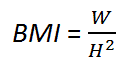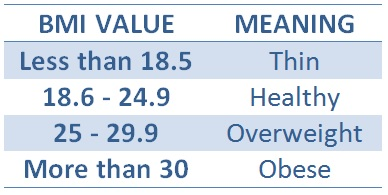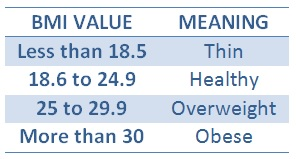How to calculate your Body Mass Index (BMI)

This article shows you how to effortlessly calculate your Body Mass Index (BMI). This is a kind of tool that compares your height and weight, and so gives you an indication of whether you are underweight, healthy or overweight.

1.- Manually Calculate your Body Mass Index using Metric Units

2.- Example with Metric Units

3.- Use Imperial Units (the American Standard)

4.- Example with American Units

5.- Online Calculator

6.- Video Summary

7.- Code a GUI using Matlab (another page within this site)

1.- Calculate your BMI using Metric Units

a) Take your weight (in kilograms, kg), we'll call this W.

b) Take your height (in meters, m), we'll call this H.

c) The BMI is easily calculatedd) This is the table that you have to look at to find out about your health. Locate the value that you just found and see its meaning. A healthy value goes from 18.6 to 24.7. Less than that means that you're thin, and more than that range means that you're overweight.2.- Example with the Metric System

If your weight is 90 kg and your height is 1.77 m, then

BMI = 90/1.772 = 28.73 and that means that you are overweight!

3.- Calculate your Body Mass Index using the Imperial (Anglo or British) System

a) Take your weight (in pounds, lb). We'll call this W.

b) Take your height (in inches, and 1 ft equals 12 inches). We'll call this H.

c) The BMI is easily calculatedd) The same table (as above) applies...

4.- Example with the Imperial System

If your weight is 198 lb and your height is 70 in, then

BMI = (198/702) x 703 = 28.4, and (see the table above) that means that you are overweight!

5.- Calculator

 To be able to use the following calculator you have to allow the use of JavaScript codes on your navigator.

 BMI Metric Units Weight = kg Height = m BMI = BMI Anglo Units Weight = lb Height = ft in BMI = Now, look at the table below to see the meaning6.- Video Summary

From 'Calculate your Body Mass Index ' to home

From 'Calculate your Body Mass Index' to Online Calculators

 Top